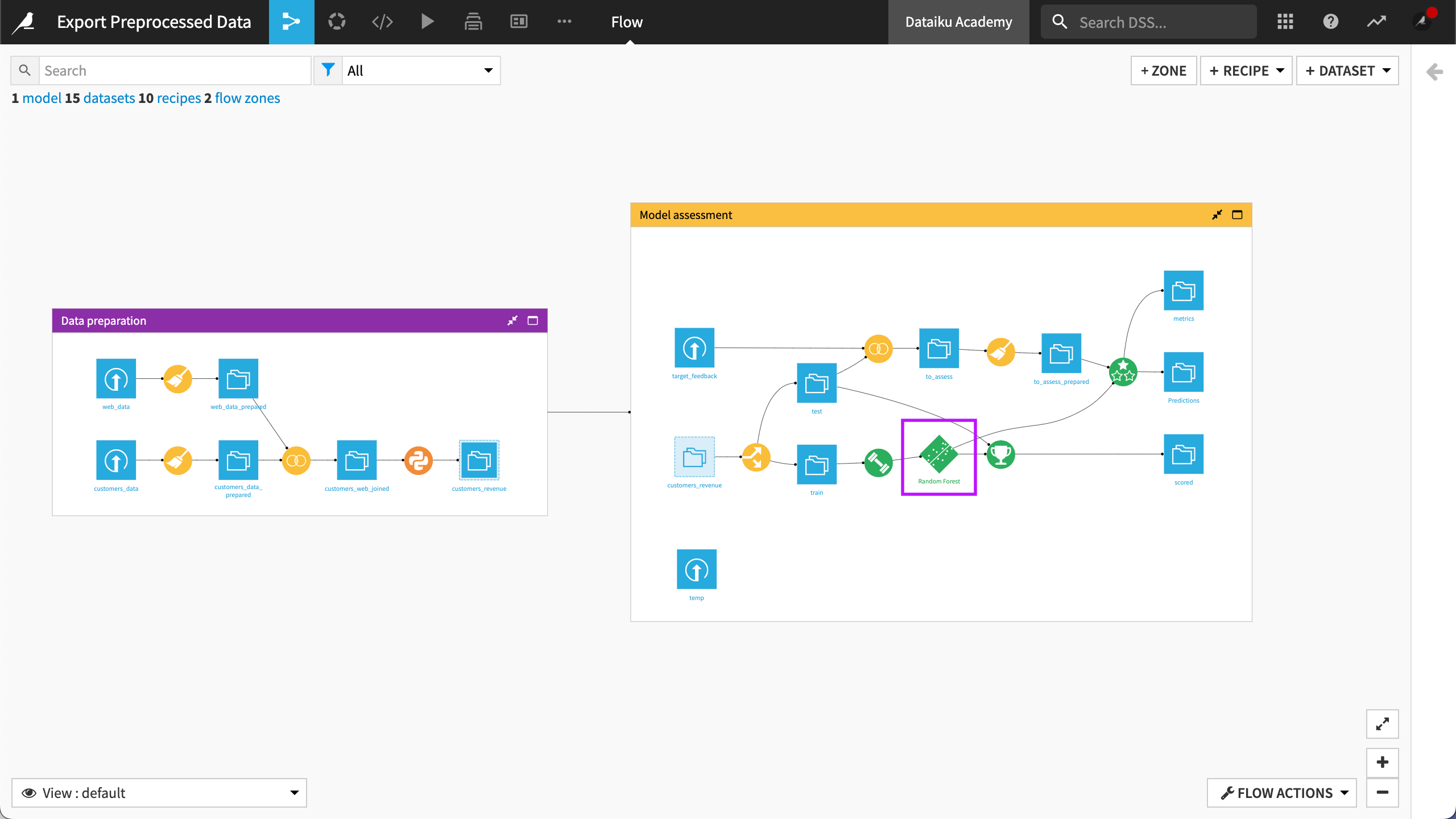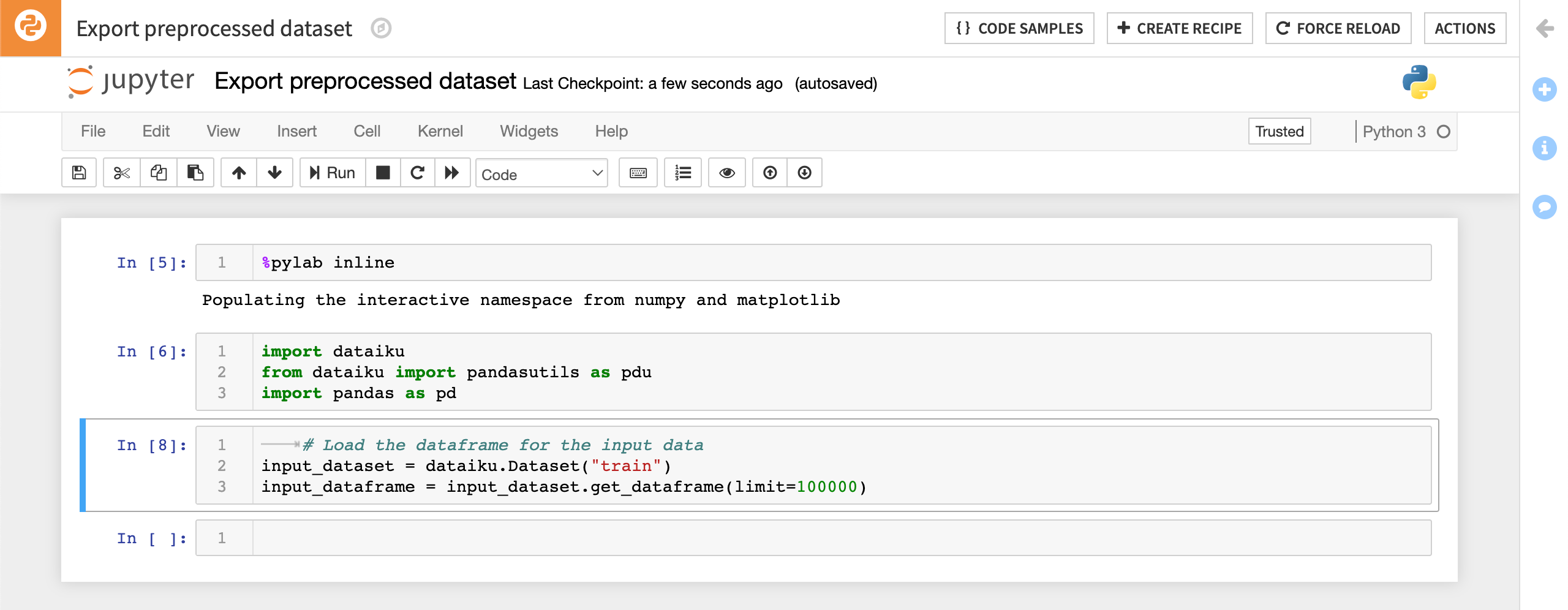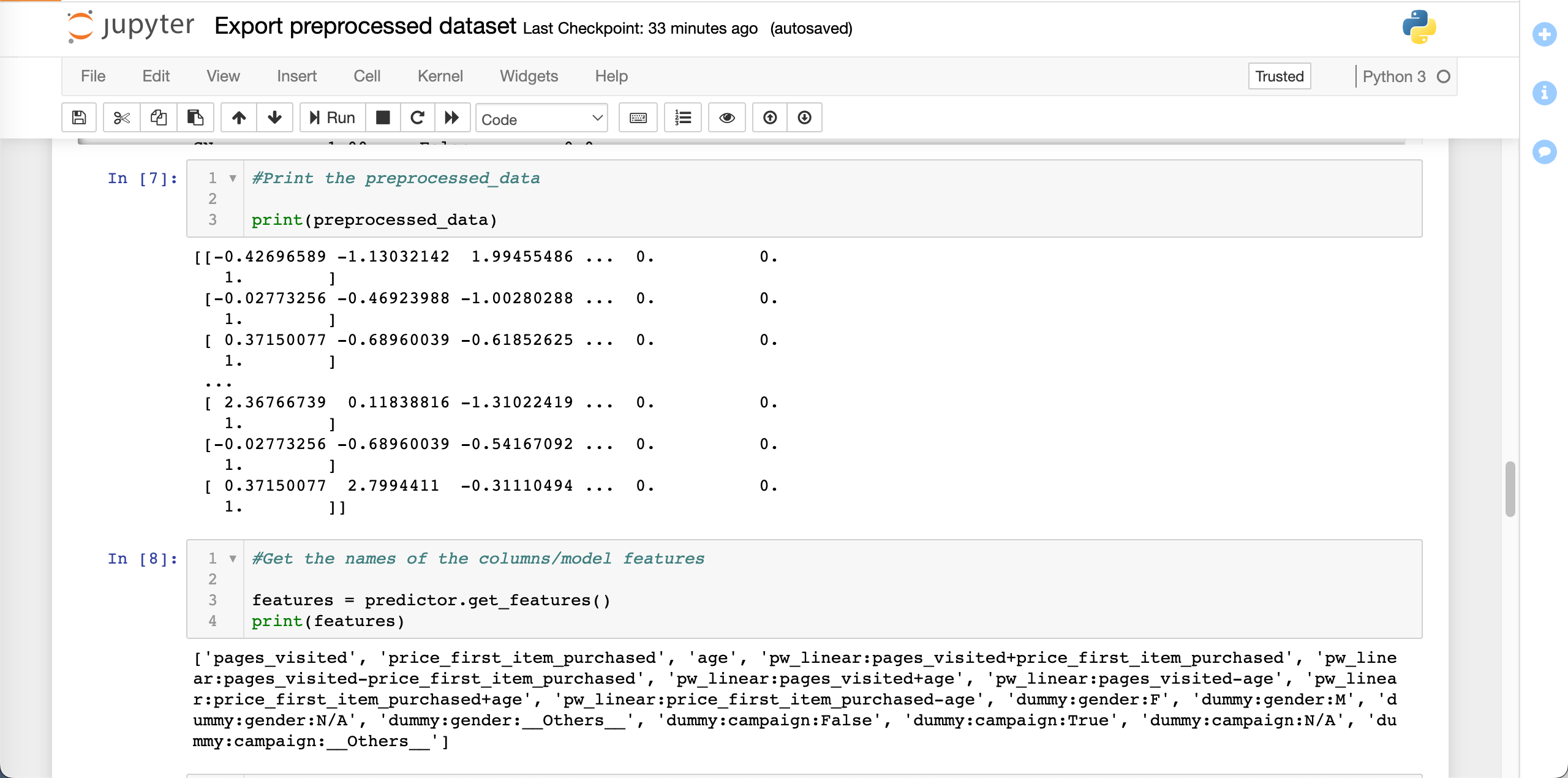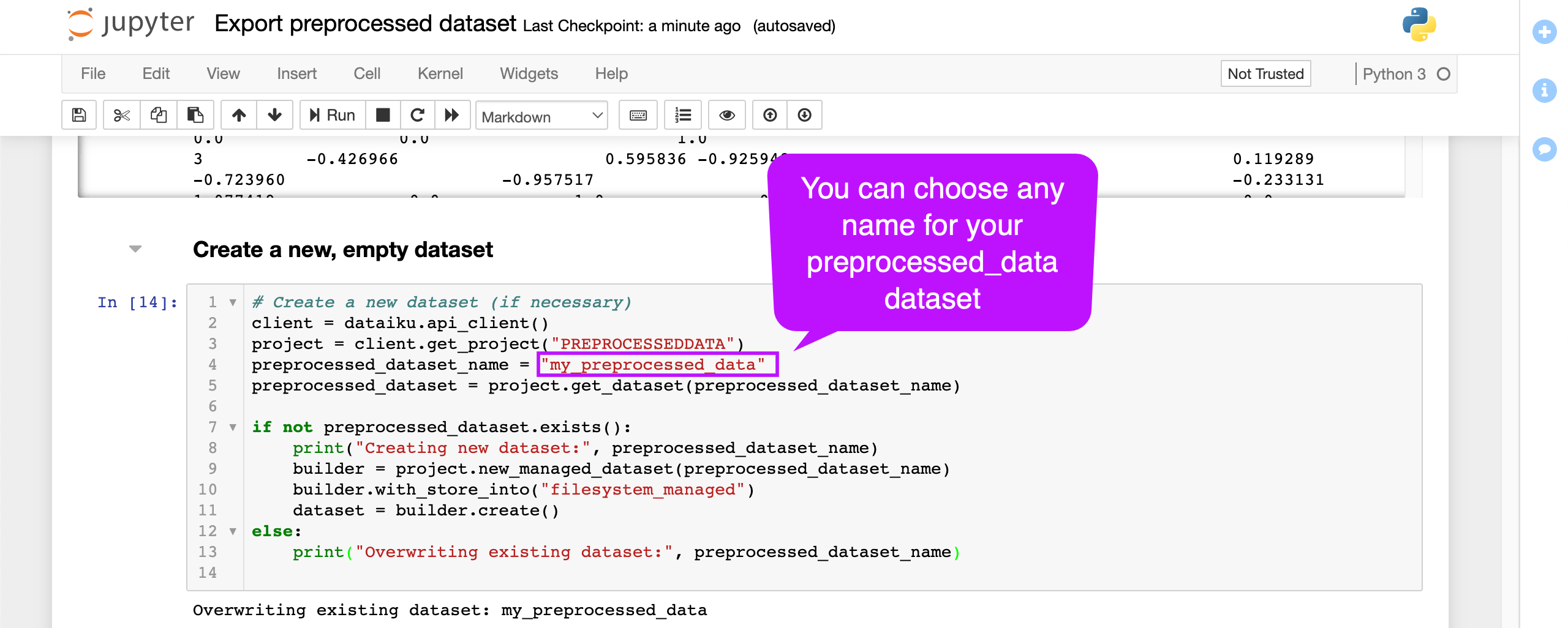# How to Export Preprocessed Data¶

To train a machine learning model, Dataiku modifies the input data you provide and uses the modified data, known as preprocessed data. You may want to export the preprocessed data and inspect it, such as when you want to investigate issues or perform quality checks.

In this article, we’ll show you how to export the preprocessed dataset using Python code in a Jupyter notebook.

Note

Dataiku comes with a complete set of Python APIs.

## Let’s Get Started!¶

In this article, we’ll work with an example of a project that contains a deployed model in the Flow. To follow along with the steps, you can use any project with a deployed model in the Flow.## Create a Code Notebook¶

From your project, create a new Jupyter notebook.

We’ll start by using the Dataiku API to get the input dataset for our model, as a pandas dataframe.

• Replace saved_model_input_dataset_name with the name of your model’s input dataset.

import dataiku
# Load the dataframe for the input data
input_dataset = dataiku.Dataset("saved_model_input_dataset_name")
input_dataframe = input_dataset.get_dataframe(limit=100000)Next, we’ll use the predictor API to preprocess the input dataframe. The predictor is a Dataiku object that allows you to apply the same pipeline as the visual model (preprocessing + scoring). For more information, visit Interaction with saved models.

• Replace saved_model_id with the ID of your saved model.

# Get the model and predictor
model = dataiku.Model("saved_model_id")
predictor = model.get_predictor()


### Preprocess the Input Dataframe¶

The model’s predictor has a preprocess method that performs the preprocessing steps and returns the preprocessed version of the data.

# Use the predictor to preprocess the data
preprocessed_data, preprocessed_data_index, is_empty = predictor.preprocess(input_dataframe)


### Examine the Dataframes¶

The original input_dataframe is a pandas dataframe containing the data from your input dataset. We can print it out to see this:

print(input_dataframe)


The preprocessed_data variable is a list of lists containing the preprocessed version of this input dataset:

print(preprocessed_data)


The names of these columns are the model features, which we can get from the predictor:

features = predictor.get_features()
print(features)


Each string in the features list corresponds to one column in the preprocessed_data. We can compare these features with the list of column names from the input dataset:

print(list(input_dataframe.columns))#### Comparing the Preprocessed Data and Input Data¶

The number of features (and so the number of columns in preprocessed_data) might be different from the number of columns in the input_dataframe; and the names of some features might be different from the column names in the input dataset. This is because the feature handling settings of your model training can remove columns from the dataset and can add new features.

In addition, the number of rows in the preprocessed data can be fewer than the number of rows in the input dataset. This is also caused by the feature handling settings used when training the model. For example, rows with an empty target value can be dropped.

The preprocessed_data_index returned by the preprocess method shows you which rows from the input dataset have been used to produce the preprocessed data.

Note

To make the preprocessed data easier to compare with the input data, we can turn it into a pandas dataframe with column headings:

import pandas as pd
preprocessed_dataframe = pd.DataFrame(preprocessed_data, columns=features)
print(preprocessed_dataframe)


If you just want to look at the preprocessed data or perform simple calculations on it, then these steps may be sufficient. However, if your goal is to perform complex analyses on the preprocessed data, you should export the preprocessed data to a new dataset in Dataiku. We’ll do this in the next section.

### Export the Preprocessed Data to a New Dataset¶

The previous steps allowed us to access the preprocessed data as a pandas dataframe in a Python notebook. This can be useful for many applications, but in order to use the full power of Dataiku to analyze the preprocessed data, we can export it to a new dataset in the Flow.

#### Create a New, Empty Dataset¶

First, we’ll create a new dataset. The following code snippet uses the Dataiku API to create a new dataset if it does not already exist. That way, we can re-run our code and overwrite the dataset with updated data.

• Replace project_name with the name of your project.

• Replace my_preprocessed_data with the name you choose for your new dataset.

# Create a new dataset (if necessary)
client = dataiku.api_client()
project = client.get_project("project_name")
preprocessed_dataset_name = "my_preprocessed_data"
preprocessed_dataset = project.get_dataset(preprocessed_dataset_name)

if not preprocessed_dataset.exists():
print("Creating new dataset:", preprocessed_dataset_name)
builder = project.new_managed_dataset(preprocessed_dataset_name)
builder.with_store_into("filesystem_managed")
dataset = builder.create()
else:
print("Overwriting existing dataset:", preprocessed_dataset_name)#### Fill the Empty Dataset with the Preprocessed Data¶

Now that our empty dataset has been created, we can fill it with the preprocessed data.

# Write the preprocessed data to the dataset
preprocessed_dataset.get_as_core_dataset().write_with_schema(preprocessed_dataframe)


This creates a new dataset in the Flow containing the preprocessed data for our model.

Note

This new dataset is not linked to your model. If you modify your original dataset or retrain your model, you’ll need to re-run the code in your notebook to update the preprocessed dataset.

## What’s Next?¶

You can now use all the features of Dataiku to analyze this dataset. For example, you can:

• Explore this dataset using the Dataiku UI, to analyze columns, compute dataset statistics, and create charts.

• Use this dataset as part of a dashboard.

• Use this dataset as the input to a recipe.

To automate updating the preprocessed dataset you could create a scenario and add a step to execute Python code. You could also create a code recipe from your code notebook.